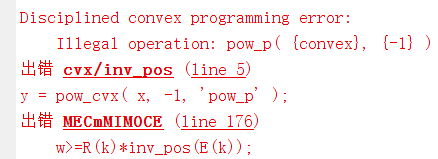# Illegal operation: pow_p( {convex}, {-1} )

Hi,everyone!
There are some problem about CVX, the matlab codes are given here:

`````` P_max_dBm=[20:1:30];
for p=1:length(P_max_dBm)
P_max=10^(P_max_dBm(p)/10-3);
cvx_begin sdp
variable f1(Number_User,1)
variable R(Number_User,1)
variable E(Number_User,1)
variable w
variable t
maximize w
subject to
for k=1:Number_User
R(k)=Rul(k)*t+f1(k)*T/C1;
E(k)=epsilon_1*pow_pos(f1(k),3)*T+t*(tao1/(tao-tao1)*P_tao1+epsilon_2*Pk0(k)+epsilon_3*C2*Rul(k)*f2^2+P_k0(k)*n(k)*u*Rul(k)/Rdl(k));
end
for k=1:Number_User
0<=Pk0(k)<=P_max;
0<=P_k0(k)<=P_max;
T*f1(k)/C1>=L;
(tao/(tao-tao1)+C2*Rul(k)/f2+u*Rul(k)/ Rdl(k))*t<=T;
end
w>=R(k)*inv_pos(E(k));
cvx_end
cvx_status
end
``````

but everytime I run this code, matlab gives messages error usingiAny help from your guys would be appreciated.

Hello and welcome to the forum.

The argument of `inv_pos` must be concave, which it is not.

help inv_pos

inv_pos Reciprocal of a positive quantity.
inv_pos(X) returns 1./X if X is positive, and +Inf otherwise.
X must be real.

`````` For matrices and N-D arrays, the function is applied to each element.

Disciplined convex programming information:
inv_pos is convex and nonincreasing; therefore, when used in CVX
specifications, its argument must be concave (or affine)
``````

Even if `inv_pos(E(k))`were “legal”, multiplying it by a variable (`f1`) lurking inside `R(k) ` would not be DCP-compliant.

In short, it is hardly evident that this problem is convex. I will assume it is not unless you show otherwise.

sorry for not replying to you yesterday. I am also posting for the first time.After reading your reply, I plan to replace multivariate multiplication with auxiliary variables. Can this be a solution?

If the problem is convex, maybe. If it is not convex, no.